# CBSE Science Notes for Class 9 Chapter 9 FORCE AND LAWS OF MOTION

CBSE Science Notes For Class 9 Chapter 9 FORCE AND LAWS OF MOTION Download Pdf

Chapter 9 CBSE Science Notes for Class 9 FORCE AND LAWS OF MOTION

## Chapter 10 GRAVITATION

Gravity is one of the most basic forces in the universe. It plays a fundamental role not only in the structure of our solar system but also in the way objects behave on Earth. In this section, we will talk about gravity on a small scale. We will discuss topics such as weight, free fall, and ballistics. We will learn the physics of phenomena we experience daily and take for granted

#### 1.Gravitation

Gravitation is the force of attraction between two objects in the universe.
Gravitation may be the attraction of objects by the earth.
This force is proportional to the product of masses of the objects and inversely proportional to the square of the distance between them. It is .independent of medium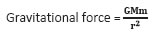Eg :- If a body is dropped from a certain height, it falls downwards due to earth’s
gravity.
If a body is thrown upwards, it reaches a certain height and then falls downwards
due to the earth’s gravity.

• Gravitation may be the attraction between objects in outer space.
Eg :- Attraction between the earth and moon.
Attraction between the sun and planets.

### GRAVITY

• A natural force that pulls all objects toward the center of the earth

• keeps the moon orbiting

• It holds stars together . . .

• And binds galaxies together for billions of years ….Prevents Planets from losing their atmospheres.

Test yourself:

1. When we move from the poles to the equator. Hence, the value of g decreases. Why?

2. What is the difference between gravity and gravitation?

2.Universal law of gravitation: - “Inverse square law”- All bits of matter attract all other bits of matter………..

• The universal law of gravitation states that, ‘Every object in the universe attracts every other object with a force which is directly proportional to product of the masses and inversely proportional to the square of the distance between them.’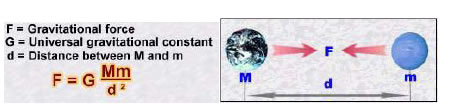• The SI unit of G is N m2 kg -2 and its value is 6.673 x 10-11 N m2 kg -2

• The strength of the gravitational attraction between two objects depends on two factors:

• How big the objects are (how much mass they have) and

• How far apart they are.

Test Yourself

1. What is the difference between gravity and gravitation?

2. What does it mean to say that the Force of gravity is proportional to the masses of the bodies, and inversely proportional to the distance between them?

#### 3. Free Fall

• With negligible air resistance, falling objects can be considered freely falling.
objects of different shapes accelerate differently (stone vs feather)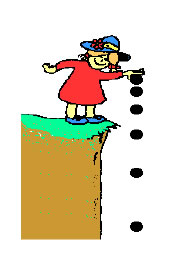#### Test Yourself

1. A coin and a feather are dropped from the roof of a building. Which one will fall to the ground first

4 .To calculate the value of” g “(acceleration due to gravity)

• The acceleration due to gravity is denoted by g.
• The unit of g is same as the unit of acceleration ms -2
• From the second law of motion, force is the product of mass and acceleration.

F = ma

• For free fall, force is the product of mass and acceleration due to gravity.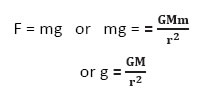where M is the mass of the
Earth and d is the distance between the object and the earth.

• For objects near or on the surface of the earth d is equal to the radius of the earth R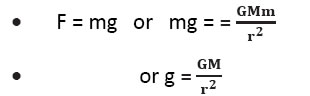Test Yourself

1. Calculate the value of g on the surface of earth.

2. What is the difference between "weight" and "mass”?

### 5. Mass and Weight:

• Mass is a fundamental, universal property. You have the same amount of mass no matter where you are in the Universe.

• Weight is not fundamental its value depends on circumstances in the Universe. Weight is a force. It is the resultant gravitational force

exerted on a body with mass m by all the other bodies on the Universe.

Weight = Fg = G m Me / R2 = mg

where Me is the mass of the Earth and R is the radius of the Earth.

Test Yourself

1. An astronaut has 80 kg mass on earth (a)what is his weight on earth? (b) What will be his mass and weight on mars where g=3.7 m/s2.2.

When you put an object on a spring balance, do you get the mass of an object or its weight?

6.Weight Of The Object On Moon

• The mass of the moon is less than the mass of the earth. So the moon exerts lesser force on the objects than the earth.

• The weight of an object on the moon is one sixth (1/6th) of its weight on the earth.

• The weight of an object on the earth is the force with which the earth attracts the object and the weight of an object on the moon is the force with which the moon attracts the object.

 Celestial body Mass(kg) Radius (m Earth 5.98 x 1024 6.37 x 106 Moon 7.36 x 1022 1.74 x 106

Test Yourself

1. What will be the weight of the body on the moon whose mass is12 kg?

### 7.Thrust & Pressure

• Thrust is the force acting on an object perpendicular to the surface.

• Pressure is the force acting on unit area of a surface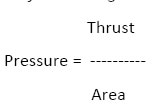• The SI unit of thrust is N/m2 or N m-2 . It is called Pascal (Pa).

8. Pressure In Fluids

• . Fluids exert pressure in all directions

• Pressure exerted on fluids is transmitted equally in all directions.

### 9. Buoyancy

When an object is immersed in a fluid it experiences an upward force called buoyant
force. This property is called buoyancy or upthrust.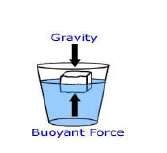10. Why objects float or sink when placed on the surface of water?

• Take some water in a beaker. Take a piece of cork and an iron nail of the same mass. Place them on the water. The cork floats and the nail sinks.

• If the density of an object is less than the density of a liquid, it will float on the liquid and if the density of an object is more than the density of a liquid, it will sink in the liquid.

11. Archeimedes Principle

• Archimedes’ principle states that, When a body is partially or fully immersed in a fluid it experiences an upward force that is equal to the weight of the fluid displaced by it.’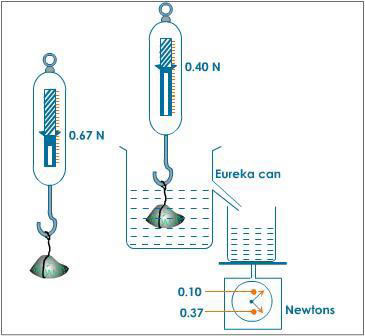12 .Relative density

• The relative density of a substance is the ratio of the density of a substance to the density of water. It is a ratio of similar quantities and has no unit.

QUESTION BANK

One Mark questions

1. Explain what Centrifugal force is.

2. What do you mean by the weight of the body on moon.

3. Give the value of G with proper units.

4. Give the value of g with proper units.

5. What is measured by physical balance?

Two Marks questions

1. At what height above the earth’s surface would the value of acceleration due to gravity
be half of what it is on the surface? Take radius of earth to be R.

2 . A body of 90 kg f on the surface of earth. How much will it weigh on the surface of
moon whose mass is 1/9 and radius is ½ of that of earth?

3. A piece of paper takes much longer to fall than a stone through the same distance.
Explain the reason.

4. Consider a heavenly body which has a mass twice that of the earth and radius thrice
that of the earth .What will be the weight of the book on this heavenly body, if its
weight on earth is 900 N?

Three Marks questions

1. Why gravitational force is usually unnoticeable?

2. Prove that acceleration due to gravity is independent of mass of the body.

3. How can the average density of the earth can be determined?

4. What is buoyancy and buoyant force? Upon what factors do they depend
Five Marks questions

1. Find the percentage change in the weight of a body when it is taken from equator to
poles. The polar radius is 6,357 Km and equatorial radius is 6,378 Km.

2. The density of ice is 918kgm-3 and that of sea water is 1,030kgm-3.An iceberg floats
with a portion 224 liters outside water. Find the volume of iceberg.

3. What are the laws of flotation? Give some illustrations.### NCERT Books Free Pdf Download for Class 5, 6, 7, 8, 9, 10 , 11, 12 Hindi and English Medium

 Mathematics Biology Psychology Chemistry English Economics Sociology Hindi Business Studies Geography Science Political Science Statistics Physics Accountancy